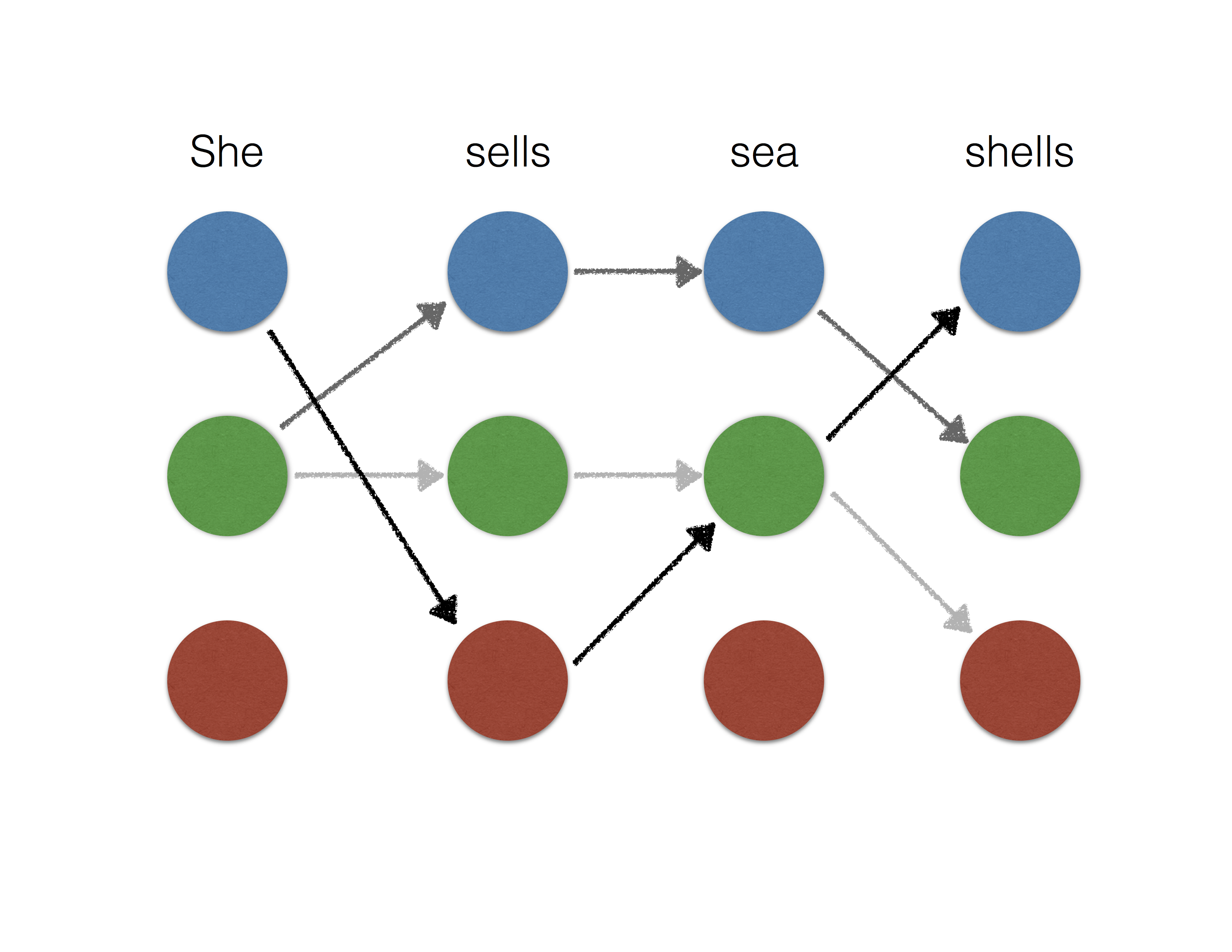Training Deep Structured Prediction Models at Scale This post discusses the use of smoothing and accelerated incremental algorithms for faster training of structured prediction models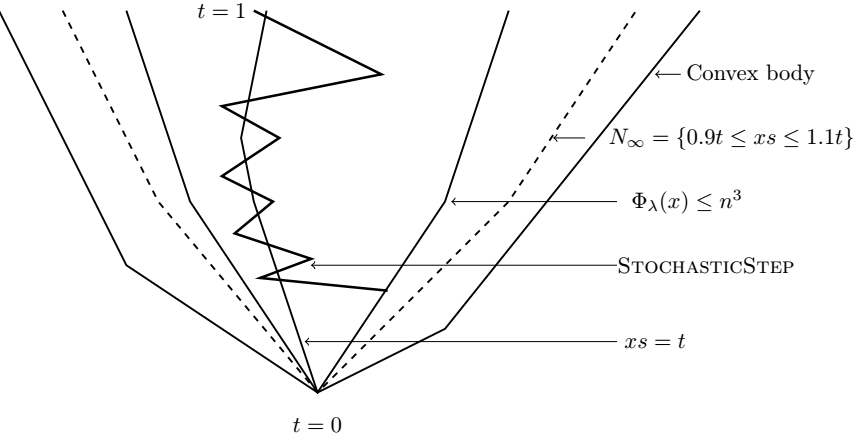Stochastic Central Path & Projection Maintenance Solving Linear Programs in the Current Matrix Multiplication Time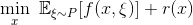Stochastic subgradient method converges at the rate $$O(k^{-1/4})$$ on weakly convex functions Recent breakthrough on using proximal stochastic gradient method for weakly convex functions.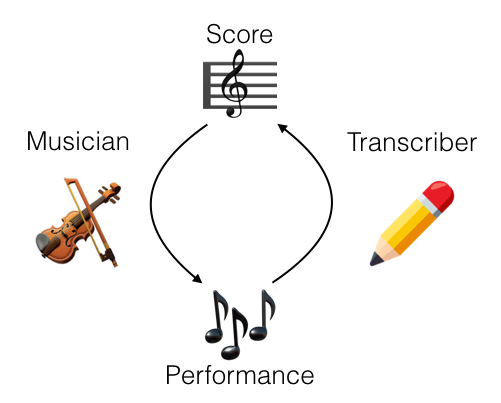Acoustic models for music transcription Recent work on music-to-score alignment and translation-invariant networks for music transcription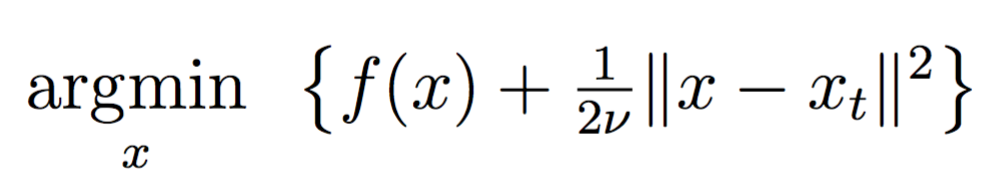Proximal point algorithm revisited, episode 3. Catalyst acceleration Revisiting the proximal point method, and catalyst generic acceleration for regularized Empirical Risk Minimization.Proximal point algorithm revisited, episode 2. The prox-linear algorithm Revisiting the proximal point method. Composite models and the prox-linear algorithm.Proximal point algorithm revisited, episode 1. The proximally guided subgradient method Revisiting the proximal point method, with the proximally guided subgradient method for stochastic optimization.The proximal point method revisited, episode 0. Introduction Revisiting the proximal point method. Introduction and Notation.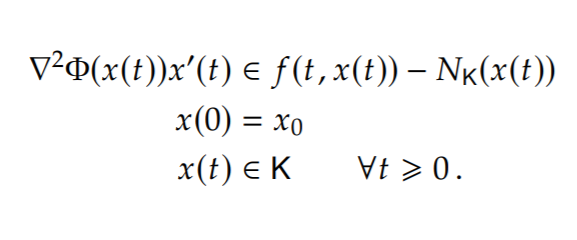k-server problem Recent breakthrough of the k-server problem on HST